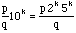Sender: Debra Subject: Fractions A college student studying elementary education asked me a question that I could not think of the correct answer. How can you look at a fraction and tell if is a terminating or non terminating decimal? Debra Hi Debra First you need to ensure that you have the fraction in lowest terms. Then look at the denominator. If the denominator has only 2's or 5's as prime factors then it is a terminating decimal. If not then it is a repeating decimal.   This is not difficult to justify. Suppose the fraction is p/q where p and q have no factors in common. If p/q, written in decimal form, terminates with k places after the decimal then moving the decimal k places to the right (multiplying by 10k) produces an integer. That isis an integer and hence any divisor of q is also a divisor of the numerator. But p and q have no common divisors so any divisor of q must be a divisor of (2k)(5k).   There is of course another kind of non-terminating decimal and that is that of an irrational number. I hope that this helps. Jack and Penny Go to Math Central To return to the previous page use your browser's back button.